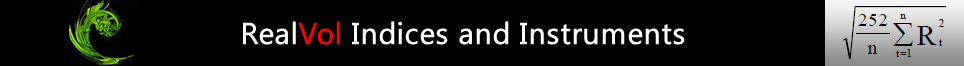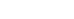# RealVol

RealVol LLC: A subsidiary of Demand Derivatives Corp. (the holding company).

# RealVol ProductsRealVol: The name used for all products from RealVol that relate to, or base their values on, realized volatility. RealVol Products can be divided into categories: RealVol Instruments, RealVol Indices, RealVol Stats, and RealVol Formulas. Within each category are the specific RealVol Products.  RealVol Instruments include: RealVol Futures, RealVol Options, RealVol Futures Options, and RealVol Swaps.  RealVol Indices include:  VOL, VOV, DVOL, VCOR, VAR, RVOL, and HVOL. RealVol Stats include PVOL, TVOL, and IVOL. RealVol Formulas include: RealVol Daily Formula and RealVol Real-Time Formula among others.

RealVol Instruments: Tradable financial instruments that settle to the 1-month (21 day) RealVol Index known as VOL

RealVol Indices: The family of daily and real-time indices that base their values on realized volatility as defined by the RealVol Formulas.

RealVol Formulas: The daily and real-time formulas that base their values on realized volatility as defined by RealVol.

vol: The generic term for volatility.

vol of vol: The generic term for the volatility of volatility.

# RealVol Instruments

RealVol Futures: Futures contracts that ultimately settle, or expire, to the appropriate VOL index.

VOL: The abbreviation/symbol for RealVol Futures.

RealVol Options: Option contracts that ultimately settle, or expire, to the appropriate VOL index. RealVol Options could also include option contracts that ultimately settle, or expire, to the associated RealVol Instrument (e.g., RealVol Options expiring to RealVol Swaps).

VOLS: The symbol for RealVol SPY Options, soon to be traded on the BOX Exchange.

RealVol Swaps: Standardized, centrally cleared, exchange-traded or over-the-counter (OTC) swaps that ultimately settle, or expire, to the appropriate VOL index.

Anticipatory Period: The first of two distinct periods over the life of a RealVol Instrument. It begins on the first day that a RealVol Instrument is listed for trading and ends on the last trading day before the start of the calculation period. Note: Not all RealVol Instruments have an anticipatory period. If the listing date coincides with the start of the calculation period, then the RealVol Instrument enters immediately into the second of the two distinct periods — the calculation period.

AP: The abbreviation for the anticipatory period.

Calculation Period: The second of two distinct periods over the life of a RealVol Instrument, and the period used by the corresponding VOL index to determine the expiration value. (Note: the ending dates of the calculation periods of individual RealVol Instruments will correspond to the associated options expiration schedule of the underlying asset or instrument.)

CP: The abbreviation for the calculation period.

# RealVol Indices

Use RealVol Indices for insight into the realized volatility of an underlying.

RealVol Real-Time Index: There is only one real-time index in the RealVol suite of risk indices. The RealVol Real-Time Index (VOL) uses a time-weighted, intra-day underlying price to provide a real-time, 21-day, realized volatility.

For instance, if the time today is 75% of the way through the current trading day, then weight the partial day's return by 75% (this partial return is self-weighting) and weight the full day's return from 22 days prior by 25% (this term needs to be specifically weighted). All other days are full-weight, full-day (close-to-close) returns. In this manner, the index encompasses 22 data points but the weight of exactly 21 trading days at any moment throughout the trading day.

Such a real-time update can be useful as a guide to position entry or exit within the trading day or for very

RealVol Daily Indices: All other RealVol Indices are daily.  This means that they are updated only once each trading day and are based only on daily observations of the underlying.  In addition, all RealVol Daily Indices come in six time frames:  1-day, 1-week (5 trading days), 1-month (21 trading days), 1-quarter (63 trading days), ), 1-half-year (126 trading days), and 1-year (252 trading days).  The six time frames correspond to the investment horizon of most traders.   For periods longer than one year, realized volatility deviates little from its very long-term average so is generally estimated to be a constant.

VOL Indices
The realized volatility indices (VOL) measure the interday (close-to-close) realized volatility of an underlying using the RealVol Daily and Real-Time Formula.  As discussed, there are five time frames for all indices including VOL.  The special flagship VOL Index is based on the 1-month time frame only.  The reason for flagship status is that the 1-month version is the only one used for tradable instruments.

VOV Indices
Realized volatility of realized volatility indices (VOV) measure the realized volatility of VOL using the RealVol Daily Formula a second time on the same data.  Trading instruments on realized volatility compels one to discover the risk of those instruments.  To do so, one needs to calculate the vol of vol.  (Note:  The second iteration through the data is always performed on a 1-month (21-day) basis, regardless of the time frame of VOL.)

DVOL Indices
The full day, overnight/intraday realized volatility indices (DVOL) measure the realized volatility based on the previous day’s close and today’s open, high, and low data of an underlying using the RealVol Overnight/Intraday Formula.  One can think of DVOL as another measure of 24-hour price risk of an underlying using additional data points for greater accuracy.

VCOR Indices
Correlation indices (VCOR) measure the correlation between the underlying and its VOL.  They are calculated by using the RealVol Correlation Formula.  Use VCOR for insight into the linear relationship between the underlying and its realized volatility.  (Note:  Two time frames, the 1-day and 1-week correlations, will not be calculated.)

VAR Indices
Realized variance indices (VAR) measure the interday realized variance of an underlying using the RealVar Daily Formula.  VAR Indices are simply the square of VOL.  One can think of VAR as another measure of interday price risk of an underlying.  Variances are easier to sum, average, and combine because the result is linear as opposed to that of volatility, which is a curve function.

RVOL Indices
The Rough Vol model (Rough Fractional Stochastic Volatility or “RFSV”) forecasts realized volatility.  According to the model, created by Professor James Gatheral of Baruch College, the log of daily high/low realized volatilities is well approximated by fractional Brownian motion with a Hurst parameter H close to zero.  RealVol uses the Rough Vol model to create the RVOL indices, which forecast the VOL index over six standardized time frames.  Our preliminary research has shown that the Rough Vol model approximates future realized volatility more accurately than the options market (implied volatility) and certain GARCH models.  Both the HARK (see below) and Rough Vol models have similar long-run predictive accuracy, but arrive at their forecasts in quite different manners — often diverging significantly over short periods of time.

HVOL Indices
HARK (Heterogeneous AutoRegressive model cast into a Kalman filter framework) is a forecast of realized volatility.  It is a dynamic extension of the asymmetric (i.e., with leverage effects) HAR model where the parameters are continuously and optimally updated by the Kalman filter according to the statistical properties of the overnight/intraday realized volatility input (DVOL). This allows flexibility and fast adaptation to the original HAR model, which was created by Professor Fulvio Corsi of Ca' Foscari University of Venice.  RealVol uses the HARK model to create the HVOL indices, which forecast the VOL index over six standardized time frames.  Our preliminary research has shown that the HARK Vol model approximates future realized volatility more accurately than the market (implied volatility) and certain GARCH models.  Both the HARK and Rough (see above) Vol models have similar long-run predictive accuracy, but arrive at their forecasts in quite different manners — often diverging significantly over short periods of time.

# RealVol Stats

Use RealVol Stats for insight into the realized volatility of a RealVol instrument.

PVOL: Partial volatility. The to-date subset of realized volatility within the CP.

IVOL: Inferred volatility. The volatility that can be inferred from the market price of a RealVol Futures (or its synthetic equivalent using option combos; i.e., long call/short put or long put/short call) and the associated PVOL.  The concept is similar to the notion of implied volatility. Implied volatility is the relative expensiveness of an option’s premium as calculated via an options pricing model and solving for the volatility input. Similarly, inferred volatility is the relative expensiveness of a RealVol Futures price obtained by applying a root-mean-square formula (perhaps more than once) to the PVOL stat and the current RealVol Futures price. The result is the aggregate of traders’ forecasts (including perhaps a risk premium) of the remaining realized volatility until expiration of the underlying asset.  Many traders would compare implied volatility to inferred volatility to judge the relative expensiveness between options and RealVol Futures, respectively.

TVOL: Theoretical volatility.  Any calculation of a theoretical value of a RealVol Futures contract based on associated options prices using the Heston Model and a root-mean-square formula.

# RealVol Formulas

Underlying Reference Prices: In regard to the RealVol Daily Formula, the once-per-day values used for the inputs to the RealVol Daily Formula. Typically, they will be the daily “closing,” “last,” “final,” or “settlement” prices of the underlying asset, instrument, index, measurement, or other daily value over a defined period. In regard to the RealVol Real-Time formula, the current, or real-time, underlying futures price will be used in addition to the daily prices just described.

URP: The abbreviation for Underlying Reference Price(s).

Realized Volatility: The actual or historical volatility that an asset or instrument displays. All realized volatilities at RealVol are based on the RealVol Formulas.

RealVol Daily Formula:A mathematical formula used to calculate the annualized standard deviation of continuously compounded daily returns of an underlying asset or instrument, assuming a zero mean, no degrees of freedom, and an annualization factor of 252 trading days regardless of the exact number of trading days in the year. The same formula is applied to three distinct concepts: (1) the general calculation of any realized volatility used by the RealVol exchange; (2) the specific calculation of any VOL index or VOV index; and (3) the specific calculation of any PVOL stat.

RealVol Real-Time Formula: All of the design elements described for the RealVol Daily Formula are the same for the RealVol Real-Time Formula. To convert from a daily to a real-time value, one needs to start with the RealVol Daily Formula, then incorporate the current underlying price (“today’s” price) and a weighting scheme. Doing so provides continuous updates throughout the trading day and delivers to traders a useful, intraday real-time indication of daily realized volatility. At RealVol, it was decided to convert only the flagship 1-month VOL (also known as VOLm) index to real-time. Essentially, the formula measures an exact 21-day realized volatility even while we are within the new, most recent, trading day.